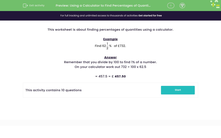# Use a Calculator to Find Percentages of Quantities

In this worksheet, students will find percentages of quantities using a calculator.Key stage:  KS 3

Curriculum topic:   Number

Curriculum subtopic:   Use Calculators/Technology for Accuracy

Difficulty level:#### Worksheet Overview

This activity is about finding percentages of quantities using a calculator.

Example

Find 62% of 732

Remember that you divide by 100 to find 1% of a number.

On your calculator work out 62 ÷ 100 x 732

= 453.84 = £ 453.84

Let's test it out in the following questions.

### What is EdPlace?

We're your National Curriculum aligned online education content provider helping each child succeed in English, maths and science from year 1 to GCSE. With an EdPlace account you’ll be able to track and measure progress, helping each child achieve their best. We build confidence and attainment by personalising each child’s learning at a level that suits them.

Get started